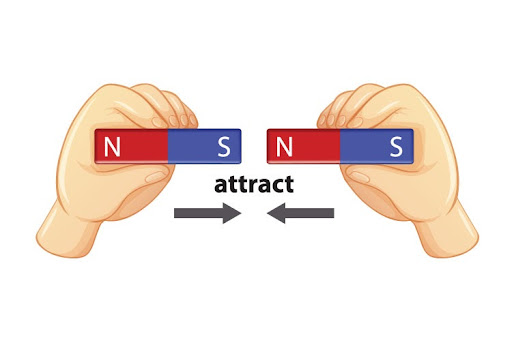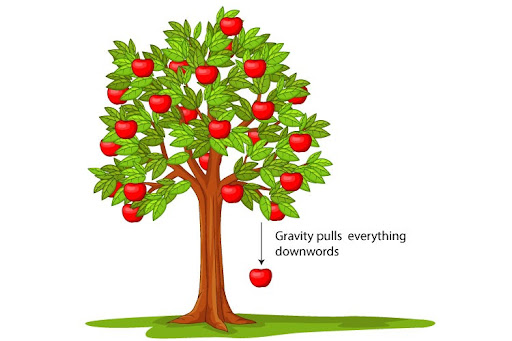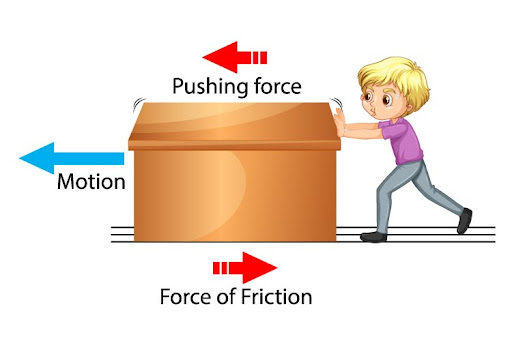# Which of the following is an example of muscular force?Two magnets attracting each otherAn apple falling from a treeCharges produced by frictionNone of these

Before we can attempt this MCQ, we would first like to define a muscular force - and that happens to be pretty self-explanatory! A muscular force is simply a force that is exerted by our muscles, arising from them contracting and relaxing upon movement. It thus becomes obvious that muscular force is a contact force - meaning it can only arise when surfaces are in contact: namely, our body and the object on which the force is being exerted.

With that out of the way, let us dive into each option!

A: INCORRECT: The scenario of two magnets attracting each other is simply a testament to the existence of the magnetic force - i.e. a non-contact force of attraction or repulsion that is exerted on moving charges due to a magnetic field. It is a non-contact force because it does not require the two magnets to be in contact in order to exist. Such a scenario does not require any muscles!B: INCORRECT: An apple falling from a tree is physical proof of the existence of gravitational force - a purely attractive force that is exerted between two masses. Thus we can explain the apple falling down simply because of the gravitational force that the Earth is exerting on it.

One may then ask: is the apple not exerting an attractive force on the Earth too? The answer to that is yes - the apple is also technically pulling the Earth up due to this mutual nature of gravitational force. But because the Earth is so massive - and the apple so small - we don’t register the Earth getting displaced.

Once again, we do not see any muscles anywhere!C: INCORRECT: Charges produced due to friction is the phenomenon wherein two surfaces rubbing against each other causes charges on the surface to be transferred, thereby creating an excess charge in one object and a lack thereof in another. Here we have to be mindful of what force is exactly causing these charges to be transferred: it is the frictional force. This is a type of contact force that resists motion, and hence exists only when two surfaces with irregularities are in contact with one another. While it is a contact force like muscular force, it does not need muscular contraction or relaxation to persist. Therefore, this too is not correct.Therefore, answer D is the best pick!